# Lecturers slides http www comp nus edu sgcs

• Slides: 13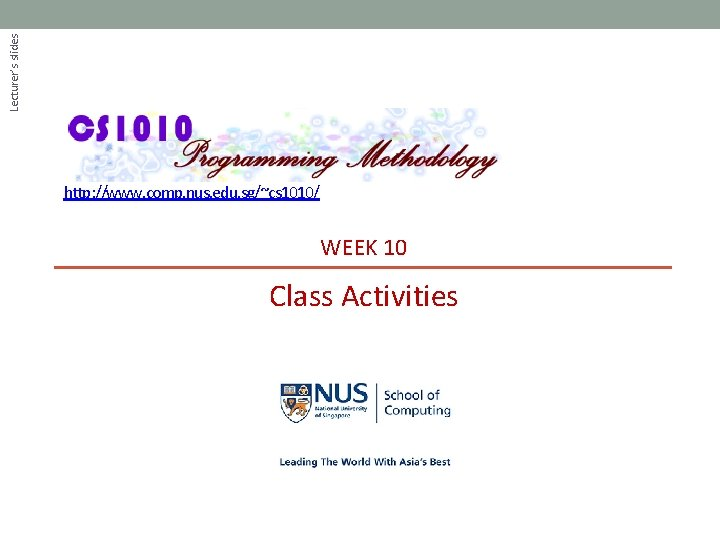Lecturer’s slides http: //www. comp. nus. edu. sg/~cs 1010/ WEEK 10 Class Activities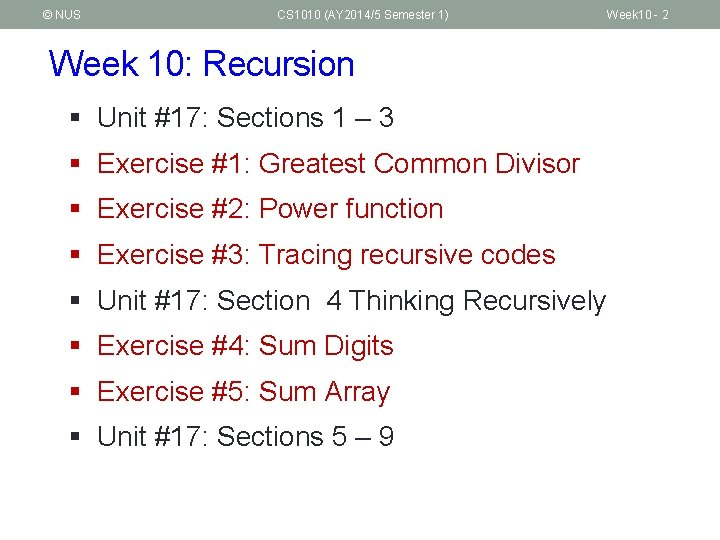© NUS CS 1010 (AY 2014/5 Semester 1) Week 10 - 2 Week 10: Recursion § Unit #17: Sections 1 – 3 § Exercise #1: Greatest Common Divisor § Exercise #2: Power function § Exercise #3: Tracing recursive codes § Unit #17: Section 4 Thinking Recursively § Exercise #4: Sum Digits § Exercise #5: Sum Array § Unit #17: Sections 5 – 9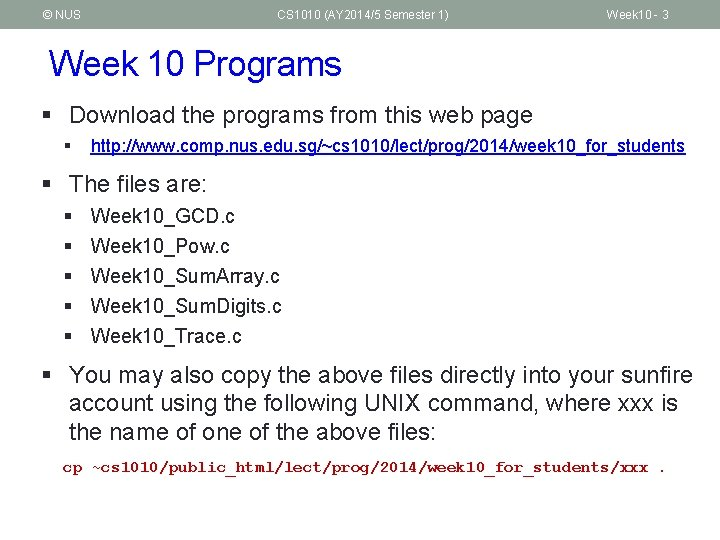© NUS CS 1010 (AY 2014/5 Semester 1) Week 10 - 3 Week 10 Programs § Download the programs from this web page § http: //www. comp. nus. edu. sg/~cs 1010/lect/prog/2014/week 10_for_students § The files are: § § § Week 10_GCD. c Week 10_Pow. c Week 10_Sum. Array. c Week 10_Sum. Digits. c Week 10_Trace. c § You may also copy the above files directly into your sunfire account using the following UNIX command, where xxx is the name of one of the above files: cp ~cs 1010/public_html/lect/prog/2014/week 10_for_students/xxx.© NUS CS 1010 (AY 2014/5 Semester 1) Unit #17: Sections 1 – 3 1. Introduction 2. Two Simple Classic Example 3. Gist of Recursion Week 10 - 4© NUS CS 1010 (AY 2014/5 Semester 1) Week 10 - 5 Exercise #1: Greatest Common Divisor § The recurrence relation for Greatest Common Divisor (GCD) of two non-negative integers a and b, not both zero, is given below: § Write a function int gcd(int a, int b) to compute the GCD of a and b. Skeleton program Week 10_GCD. c is given.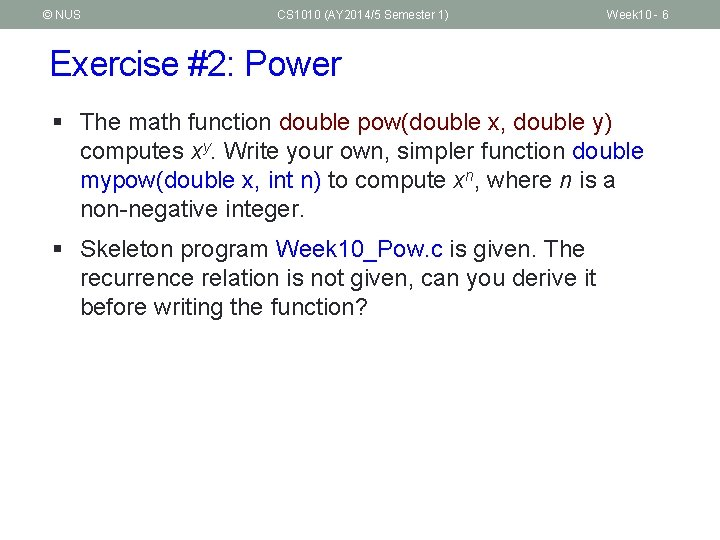© NUS CS 1010 (AY 2014/5 Semester 1) Week 10 - 6 Exercise #2: Power § The math function double pow(double x, double y) computes xy. Write your own, simpler function double mypow(double x, int n) to compute xn, where n is a non-negative integer. § Skeleton program Week 10_Pow. c is given. The recurrence relation is not given, can you derive it before writing the function?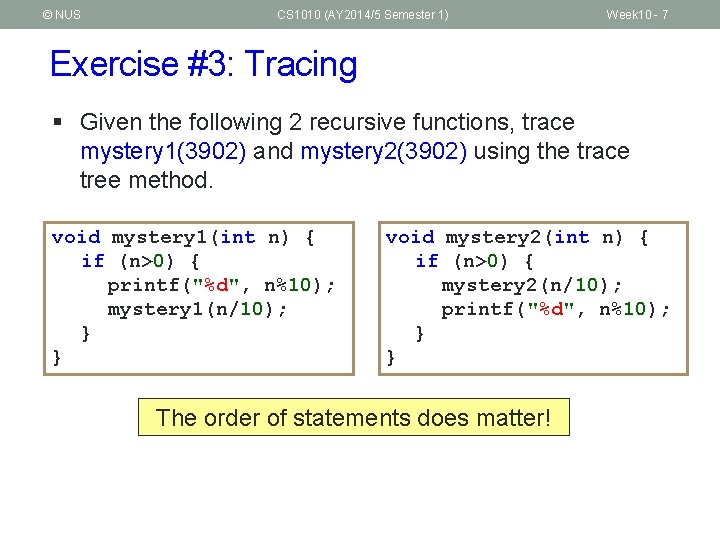© NUS CS 1010 (AY 2014/5 Semester 1) Week 10 - 7 Exercise #3: Tracing § Given the following 2 recursive functions, trace mystery 1(3902) and mystery 2(3902) using the trace tree method. void mystery 1(int n) { if (n>0) { printf("%d", n%10); mystery 1(n/10); } } void mystery 2(int n) { if (n>0) { mystery 2(n/10); printf("%d", n%10); } } The order of statements does matter!© NUS CS 1010 (AY 2014/5 Semester 1) Unit #17: Section 4 Thinking recursively Week 10 - 8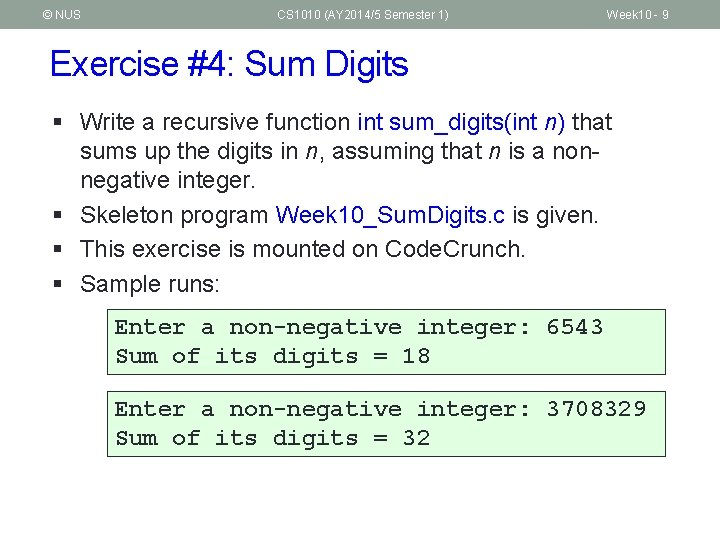© NUS CS 1010 (AY 2014/5 Semester 1) Week 10 - 9 Exercise #4: Sum Digits § Write a recursive function int sum_digits(int n) that sums up the digits in n, assuming that n is a nonnegative integer. § Skeleton program Week 10_Sum. Digits. c is given. § This exercise is mounted on Code. Crunch. § Sample runs: Enter a non-negative integer: 6543 Sum of its digits = 18 Enter a non-negative integer: 3708329 Sum of its digits = 32© NUS CS 1010 (AY 2014/5 Semester 1) Week 10 - 10 Exercise #5: Sum Array § Write a program Week 10_Sum. Array. c to read data into an integer array with at most 10 elements, and sum up all values in the array, using a recursive function. § This exercise is mounted on Code. Crunch. § Sample runs: Enter number of elements: 6 Enter 6 values: 4 3 -2 0 1 3 Array read: 4 3 -2 0 1 3 Sum = 9 Enter Array Sum = number of elements: 8 8 values: 11 25 56 8 12 7 31 16 read: 11 25 56 8 12 7 31 16 166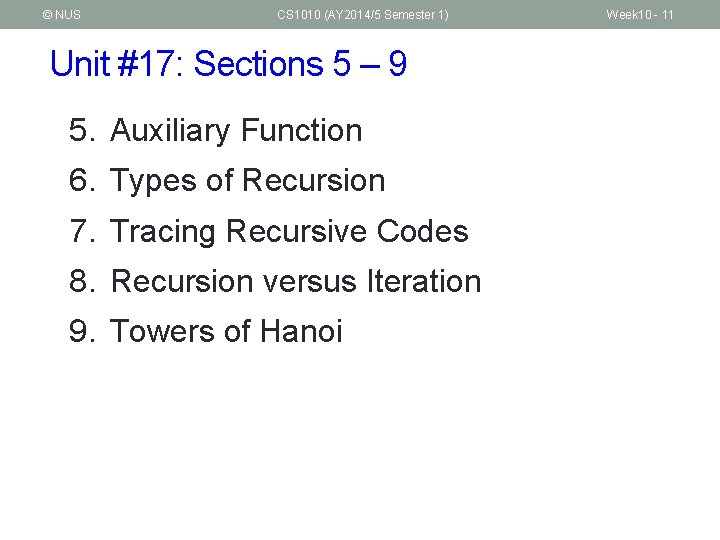© NUS CS 1010 (AY 2014/5 Semester 1) Unit #17: Sections 5 – 9 5. Auxiliary Function 6. Types of Recursion 7. Tracing Recursive Codes 8. Recursion versus Iteration 9. Towers of Hanoi Week 10 - 11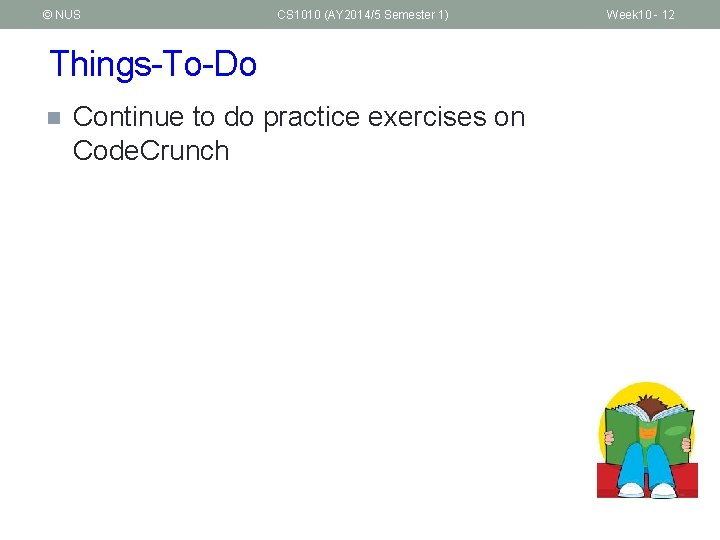© NUS CS 1010 (AY 2014/5 Semester 1) Things-To-Do n Continue to do practice exercises on Code. Crunch Week 10 - 12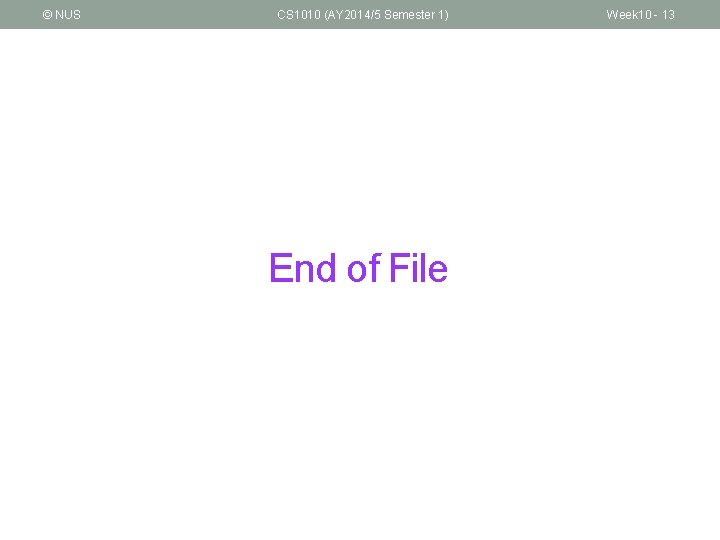© NUS CS 1010 (AY 2014/5 Semester 1) End of File Week 10 - 13